## Objective

Use System Identification Toolbox of MATLAB to estimate the transfer function of a system - given sampled data of the system’s input and output.
Then use the minimum mean square error (MMSE) estimator method to alternatively estimate the model of the system.

## Theory

Mathematical modelings importance in various fields cannot be understated. It is used to describe a system using mathematical concepts and language. When we have an interest in performing tests on a complicated system which is difficult to physically obtain, expensive, and sensitive to failure. Then it is safer and cheaper to perform the same tests on the model using computer simulations rather than carry out repetitive experimentations and observations on the real system.

In making these “Models” we have various types,

#### Theoretical Models

These models are obtained from fundamental principles, such as the laws of conservation of mass, energy, and momentum along with other chemical principles such as chemical reaction kinetics and thermodynamic equilibrium, etc.

#### Empirical models

These models are based on experimental plant data. These models are developed using data fitting techniques such as linear and nonlinear regression.

We will be dealing with empirical models in the remainder of this experiment, models obtained exclusively from experimental plant data are also known as black-box models . Such models do not provide detailed description of the underlying physics of the process. However, they do provide a description of the dynamic relationship between inputs and outputs. Thus they are sometimes more adequate for control design and implementation.

Thus we come to the issue of Parameter Estimation, given a set of input output data pairs we need to estimate the values for the coefficients used in the model such that the model best fits the experimental evidence.

Let the relationship between input and output of the system be modeled as the difference equation given below,

where $y_k$ is the $k^{th}$ output sample and $u_k$ is the $k^{th}$ input sample.

So for $n$ samples of input-output data pairs, we can write the set of simultaneous equations in matrix form in order to form a concise notation.

Consider a $(n\times1)$ vector $Y$ having elements from $y_1$ to $y_n$,
and a $(n\times2)$ matrix $\Phi$ having elements from $y_0$ to $y_{n-1}$ as the first column and $u_0$ to $u_{n-1}$ as the second column,
and finally a $(2\times1)$ vector $\theta$ which holds the two parameters $a$ and $b$.

#### MMSE Derivation

So the $n$ equations formed from entering the n data pairs into the difference equation given above can be written in matrix form as follows,

even though we write a equals sign, it is usually not possible to find a $\theta$ which perfectly models the system. we just aim for a $\theta$ such that the model most faithfully fits the given data ($n$ samples). Note that as $lim_{n \to \infty} Y = \Phi \theta$, (infinite number of data points) until then R.H.S and L.H.S continues to approach each other.

From the minimum mean square error (MMSE) estimator method, we get the following formula to calculate this $\theta$,

I will now detail the proof for the above equation which is used to estimate $\theta$ (i.e. the values of $a$ and $b$).

As I said before we need to find a $\theta$ such that the model most faithfully fits the given data, how can we make this statement into a relationship involving the parameters of interest?

So “most faithfully” can be thought of to mean, we want to minimize the error. Let us then define the error of the model as follows,

Now we don’t want to minimize this error - $E$ for all data samples. Because we care about the deviation of $O_{Models-Output}$ from $O_{Systems-Output}$, we do not care about the direction of this deviation. This is because positive and negative error of subsequent samples can cancel each other out. Thus we should take the $|E|$ - so that we have the absolute value of Error. We would rather define the error as the value $P$ (also known as Mean Square Error) rather than just $|E|$.

The reason why we prefer MSE (squaring) to taking modulus when we want to quantify this error, is due to the fact that while both eliminate the primary problem of “positive and negative error”; squaring makes the algebra much easier to work with and offers properties that the absolute method does not. Additionally the squared difference has specific mathematical properties; such that it’s continuously differentiable (which nice when you want to minimize it - like now). There are additional reasons which can be found here.

Okay getting back to our hunt for the best fit for the given data, we need to minimize $P$ with respect to $\theta$, for that we differentiate $P$ with respect to $\theta$ and equate that to zero.

Lets expand $P$,

Opening the brackets, note that $(\Phi \theta)^T = \theta^T\Phi^T$

Vector Calculus Side Note

Here both $x$ and $a$ are vectors while $A$ is a matrix.

You can skip the following proof

Now the formulas given above can be understood by the fact that vectors are just used to hold multiple elements together - for the sole reason of applying operators onto them in bulk.

So in the first formula both $a$ and $x$ are $n\times 1$ vectors. What both $x^Ta$ and $xa^T$ will be is a scalar value which is the dot product of both vectors.

Differentiating a scalar w.r.t a vector is defined as a new vector with its $i$th element being the differentiation of that scalar with the $i$th element of the vector.

Two competing notational conventions split the field of matrix calculus into two separate groups. The two groups can be distinguished by whether they write the derivative of a scalar with respect to a vector as a column vector or a row vector.

For the second formula, the proof is straightforward given the above result. Here $A$ is a $n \times n$ matrix. Apply product rule, note that the terms in the bracket are considered as constants when differentiating.

From the first formula - when comparing, $(x^TA)_{1 \times n}$ can be thought of as $a^T$ while $(Ax)_{n \times 1}$ as $a$. So,

When we partially differentiate P w.r.t $\theta$, only $\theta$ is a variable while everything else is considered to be a constant.

Partially differentiating w.r.t $\theta$ and equating to 0 we get the value of $\theta$ for which the Mean Square Error is minimum,

Here $\Phi^T \Phi$ is a symmetric matrix thus,

Moving all terms without $\theta$ to one side,

So post-multiplying both sides with $( \Phi^T \Phi)^{-1}$,

Taking transpose on both sides, note that $(( \Phi^T \Phi)^{-1})^T = (( \Phi^T \Phi)^{T})^{-1}$ because ${(A^{-1})}^T= {(A^T)}^{-1}$ and $(AB)^T = B^TA^T$.

Thus we get our $\theta$,

## Simulation

I will first generate “data” using the simulink library in MATLAB. There we can construct a model of a system and run simulations on it.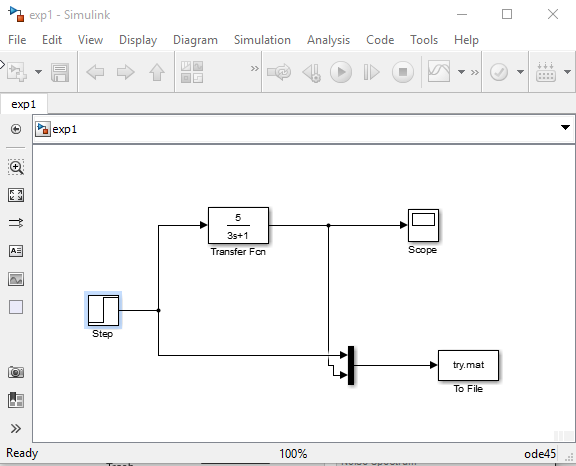Here as you can see I am applying a step input to a first order system, and writing both the input (Step) and output to the file try.mat, now looking at the settings of the step function and the ‘write to file’ block you can see I have chosen a Sampling Rate of 0.1 secs and initial time is 0 secs.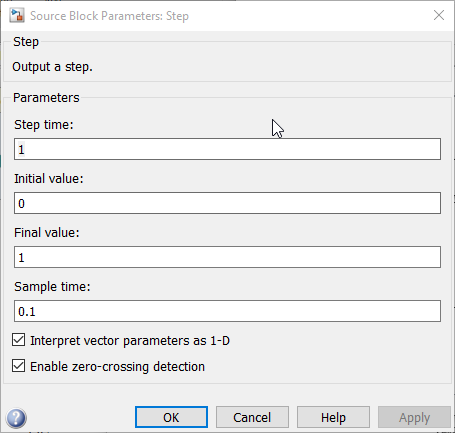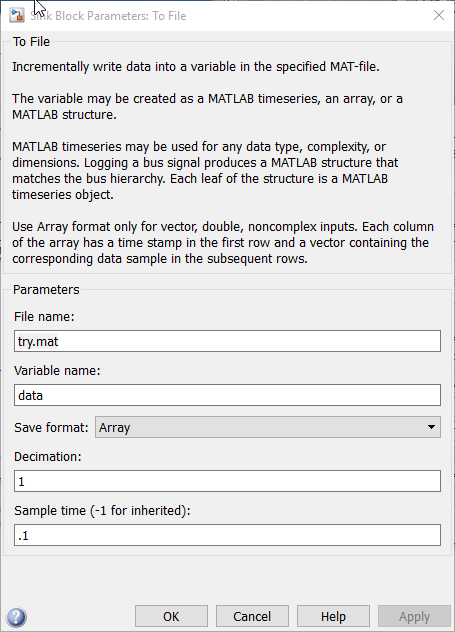So we store time, input, and output into a variable - data and this file is called try.mat. Note that as we have 0.1 sec sample time we sample every 0.1 secs for 30 secs. Therefore we will have 301 samples ($\frac{30}{0.1}$ and an initial 0 data sample).

Here the transfer function of the system used to generate the data is ,

You might get a error - Error encountered in block ‘exp1/To File’ - Error opening or closing file ‘try.mat’ . The error will open the Diagnostic Viewer as shown below,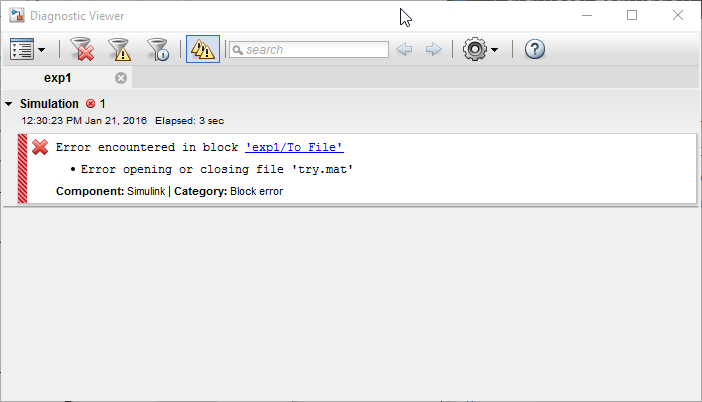We can fix this error by opening MATLAB as administrator, this error is caused due to MATLAB not having the permission to write to disk. Open MATLAB as shown in the image below,On opening the Scope block we will see the input and output superimposed on a graph with amplitude as a function of time. The yellow line is the unit step input and the purple line is the systems output.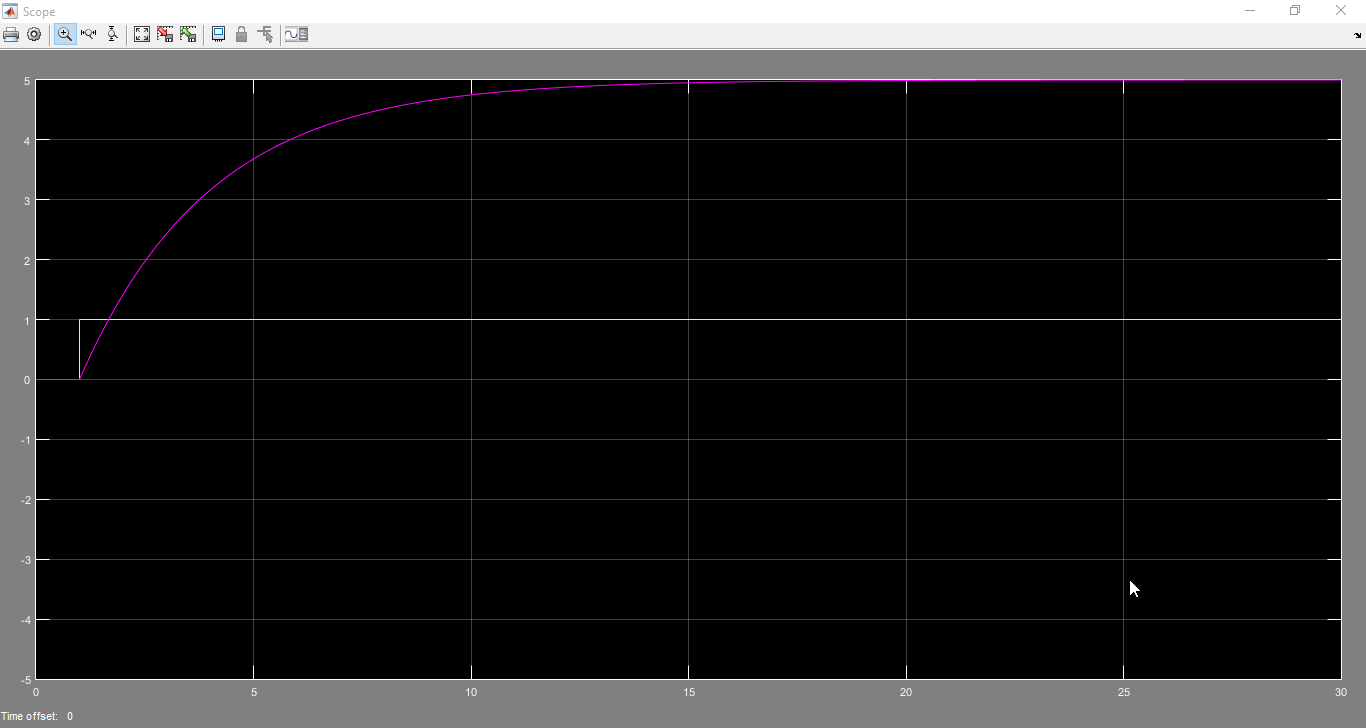In order to do that we need to load this generated data into the MATLAB’s workspace. We use the load try.mat command to achieve this, once we do that the data variable holding the time, input, and output as rows will be added to the MATLAB workspace. As you can see below the first row is the time sampled every 0.1 secs, the second row is the step input which starts at 1 sec and finally the system’s output is the third row in the data variable. Note that I printed data' for better readability that is why the rows I refer to are shown as columns in the image below.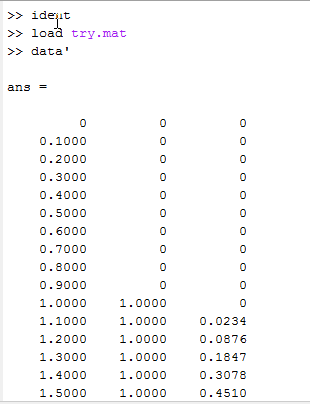This data variable can now be separated into its 3 components using the commands as shown below,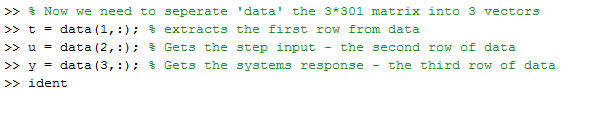We will be now using the t, u, and y variables we have made in order to reverse engineer the above system transfer function.

### The Minimum Mean Square Error (MMSE) estimator method

The first method is using the equation we just derived which models the system using a first order difference equation, and then minimizes the Mean Square Error between the actual system’s output and the model’s predicted output.

Now that we have the time, input, and output variables in the workspace, we can construct $Y$ and $\Phi$ using the commands as shown below,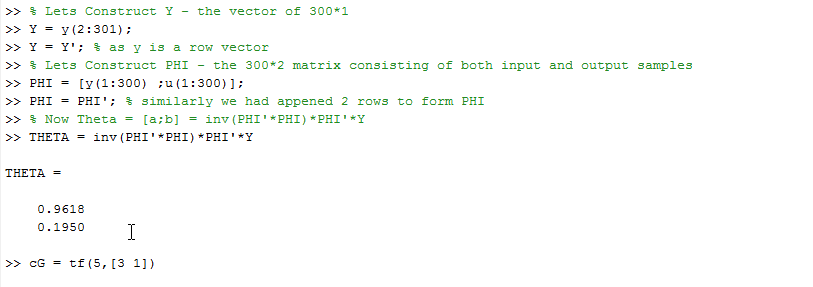Note that in MATLAB element’s indexes range from 1 to n rather than 0 to n like more programming languages.

As I had explained in the proof, I am taking the all but the first data element when I construct $Y$, and all but the last element when i construct $\Phi$.

Then I apply the formula to find the $\theta$ for which the MSE is minimum. This comes out to be for values of $a$ = 0.9618 and $b$ = 0.1950.

Let us now verify how well these parameters fit the actual system, for this I am using MATLAB’s c2d function to take my continuous time i/o system and find its equivalent discrete time i/o system with a sampling time of 0.1 secs (Just like the data we generated).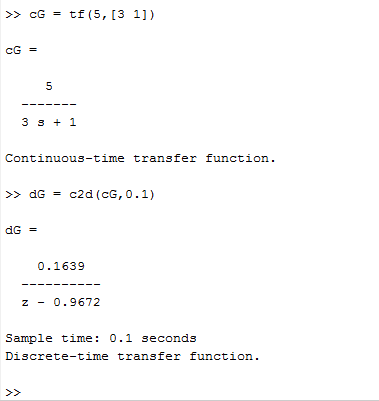Note that the $dG$ shown in the image is a approximation of $cG$, and with smaller sampling time this approximation eventually tends to become the continuous-time model itself!

For better understanding I will elaborate on how the $dG$ tells us what $a$ and $b$ are. MATLAB tells us,

Cross multiplying, we get

By the Time shifting property of Z transforms i.e. $z^{-k}X(z) = x[n-k]$,

Doesn’t the above equation look familiar? yes it is of the same structure as the difference equation we used to model this system. Just as first order systems are modeled using $\frac{k}{\tau s + 1}$, we can use the difference equation of this form to model the equivalent discrete time model system.

Thus the best fitting which MATLAB performs using its “inbuilt algorithms” (which are based on the same mathematics we used, but has multiple small modifications to improve its speed and accuracy) is $a$ = 0.9672 and $b$ = 0.1639.

So an error of $\delta a$ = 0.0054 and $\delta b$ = -0.0311 seems acceptable.

### System Identification Toolbox

We can also use the System Identification Toolbox that comes with MATLAB to estimate the same parameters. This method has the additional benefits in the fact that they have added options for all algorithms to estimate the parameters, runs extremely fast, graphs the model formed etc.

We can use ident to open the System Identification App, we will see a window as shown below open up,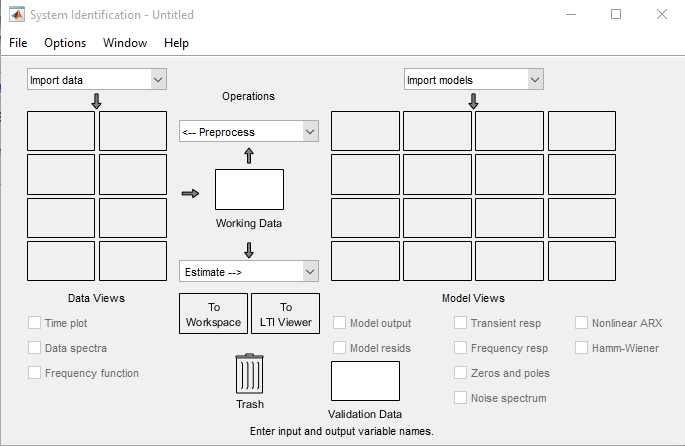We can now import the data using which MATLAB will estimate the parameters,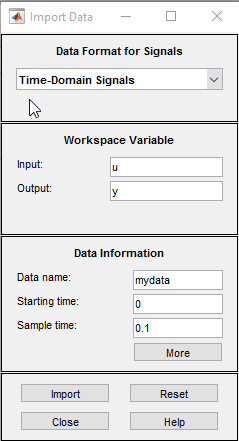Note that we need to keep the sampling time as 0.1 sec and starting time as 0 secs just as we defined it in the simulink model. Now click import to get the data into ident. The time domain data will look like the below image,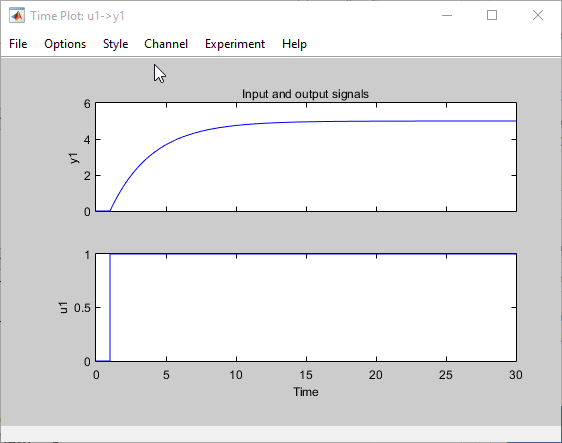Now using Estimate -> Transfer Function Models, we are prompted for number of poles and zeros which we need to enter.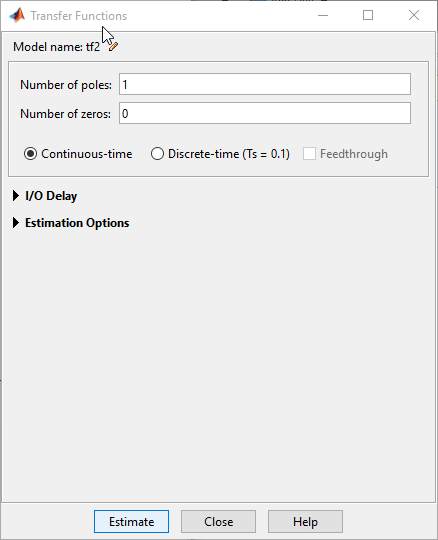After which they estimate using Instrument Variable approach (which is the default) as shown below,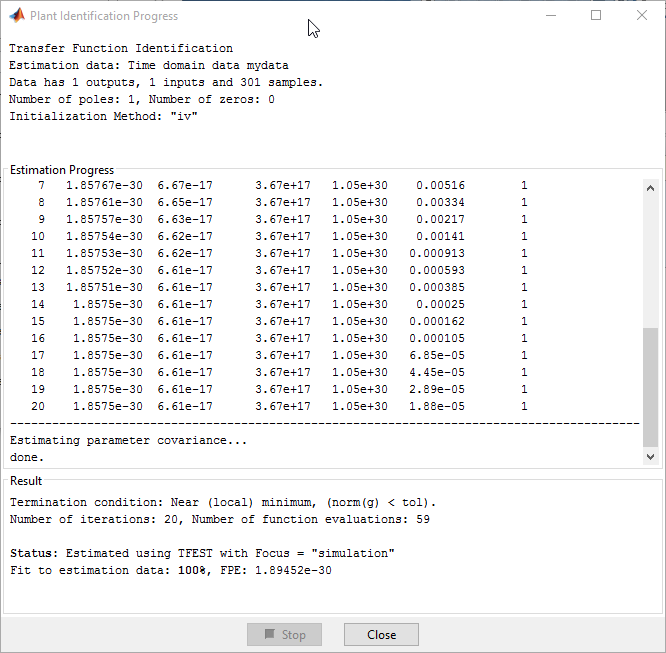Now as you can see the Transfer function has been estimated with a 100% fit.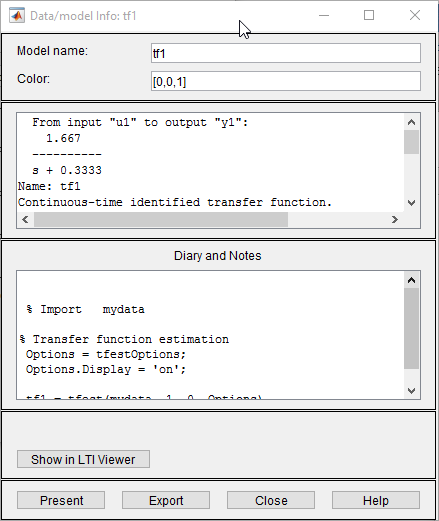This is absolutely correct as $\frac{5}{3}$ is 1.666 and $\frac{1}{3}$ is 0.333.

We can even use the “Show in LTI” option as shown in image above to see the out of a LTI system modeled on a $G(s) = \frac{\frac{5}{3}}{s+ \frac{1}{3}}$.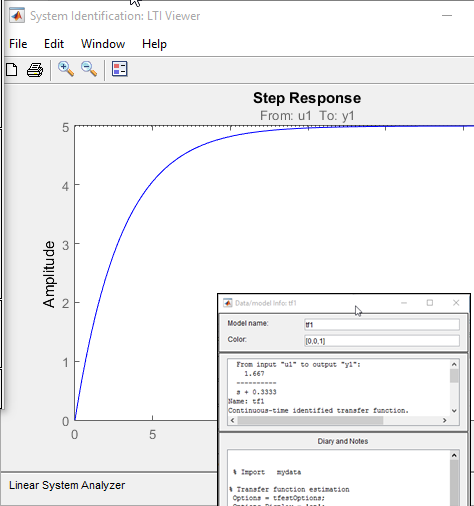There is also a different MATLAB option to do the estimation, that is using
Estimate -> Process Models,

Just untick the ‘Delay’ checkbox, uncheck ‘Zero’ checkbox - as there are no zero’s, choose the correct number of poles - 1 from the dropdown menu. Then click on estimate - We get the results quite fast,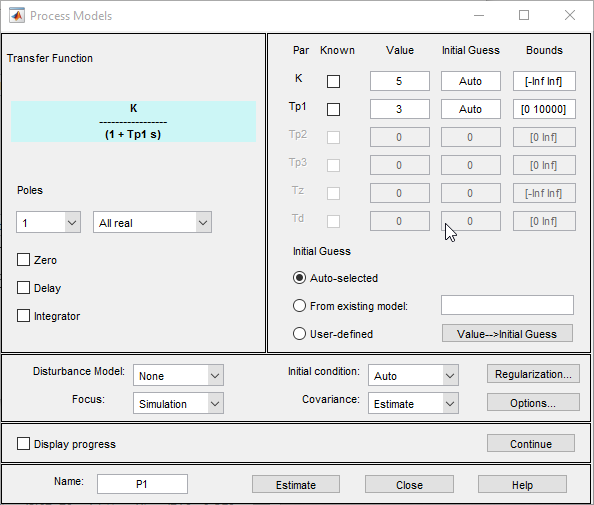As we can see the transfer function has been recovered from the time domain data here, using the $\frac{k}{Tp_1 s + 1}$ model. Where $k$ is 5 and $Tp_1$ is 3.

### Conclusion

In conclusion we have used the experimental plant data (Which we generated using simulink) to estimate black-box models . We understood the mathematics behind this generation and also employed more suitable methods available in MATLAB in the process of generating said models.

We can show very clearly the benefits of empirical models when we model the FET amplifier circuit - voltage divider bias configuration.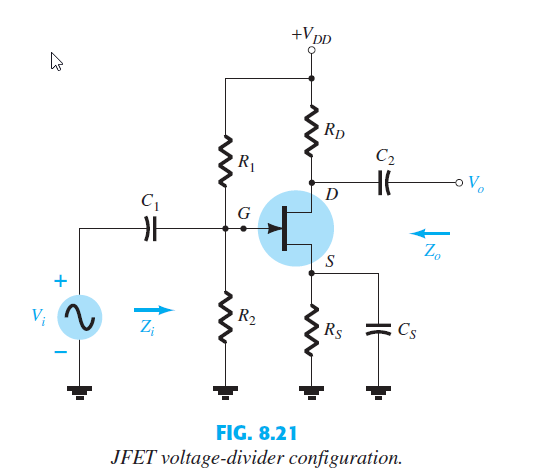Now before we begin, let’s remember that a DC gate-to-source voltage controls the level of DC drain current through a relationship known as Shockley’s equation.

So we can express the change in drain current that will result from a change in gate-to-source voltage by using the transconductance factor $g_m$.

Now substituting the ac equivalent model for the JFET, we get the following circuit,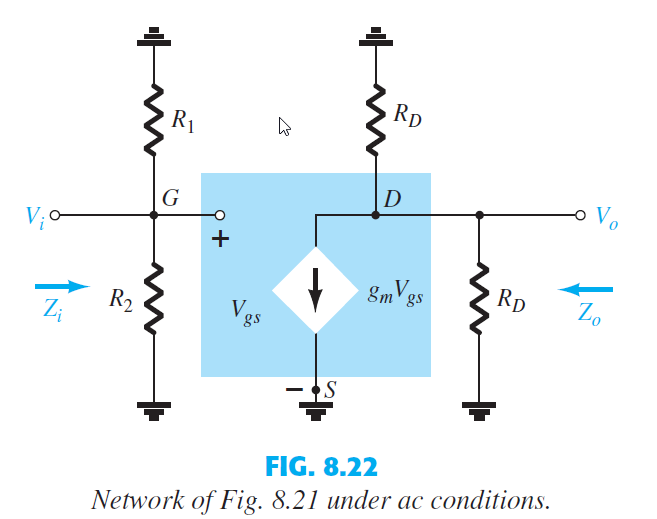From the above circuit we find the input impedance $Z_i$ and output impedance $Z_o$ as,

Setting $V_i$ = 0 Volts sets $V_{GS}$ and $g_m V_{GS}$ to zero, also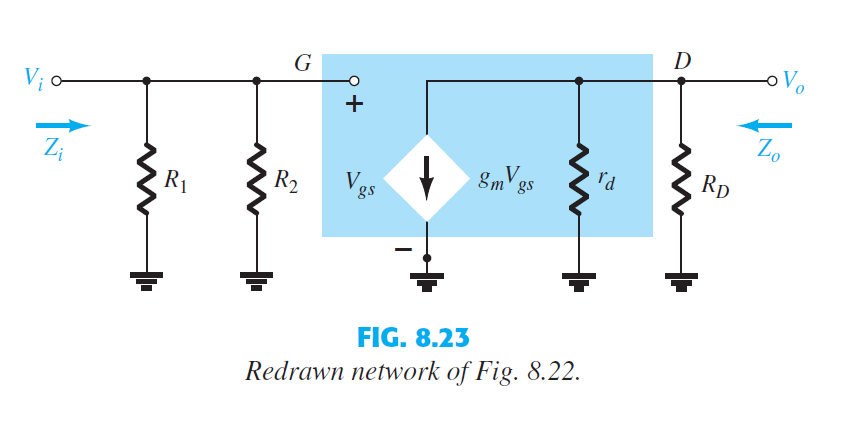So the Gain of the amplifier $A_v$ is,

The analysis thus far has been limited to a particular frequency. For the amplifier, it was a frequency that normally permitted ignoring the effects of the capacitive elements, reducing the analysis to one that included only resistive elements and sources of the independent and controlled variety.

The junction capacitance (input capacitance) $C_m$ will be virtually increased due to Miller’s effect.

where $-A_v$ is the gain of the amplifier and $C$ is the feedback capacitance.

So now the Transfer function is given by,

Now just like how we obtained the above transfer function by first principle we can use the parameter estimation methods detailed in the sections above to obtain the same transfer function.

Thus knowing that FET amplifier has a first order transfer function characteristics lets us model it using $\frac{k}{\tau s + 1}$.

Note that this is not a complete black box model, it is infact a grey box model as we know the structure (First order).

Written with StackEdit.